## Introduction

Optical communications in both free-space optical (FSO) and fiber optic technologies have achieved dramatic increments in their aggregate transmission rates using multiplexing/demultiplexing (MUX/DEMUX) technologies.1 In addition to the multiplexing technologies based on amplitude, wavelength2,3,4 and light polarization5,6 division, the orbital angular momentum (OAM) of optical vortex (OV) beams7,8,9,10,11 provides a new degree of freedom for multiplexing to further increase the capacity of optical communications systems. OV beams have helical phase profiles in their electric fields that are proportional to the azimuthal phase term of exp(i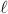φ), whereis the eigenvalue of the OAM or the so-called topological charge and φ is the azimuthal angle. In principle, the values ofare unlimited, which makes it possible to use the OAM states as information channels to carry signals12,13,14,15 for data transmission. The superior security advantages of OAM channels have also been demonstrated in both classical and quantum communication systems.14,15,16,17,18,19

OAM-based optical communications can be classified into two different categories: OAM shift keying (OAM-SK) and OAM division multiplexing (OAM-DM). For OAM-SK, the OAM states of the OV beams are regarded as a modulation format that enables the encoding of information by dynamic switching of spatial light modulators.7,19,20,21,22 For OAM-DM, the OAM states are considered to be fixed channels to be used to carry information with the advantages of high bit rates and low bit error rates (BERs).13,23 In a state-of-the-art demonstration of an OAM-based FSO communication system, 2.56 Tbit s−1 data transmission was obtained by the multiplexing of four OAM beams with two polarization states.13 Recently, the same research group achieved a data capacity of 100 Tbit s−1 through 1008 channels by multiplexing 42 wavelengths, 12 OAM beams and two polarization states together.24 However, in all the pioneering work described above, the maximum number of OAM channels that carried independent signals was only four, which is mainly constrained by the sophisticated experimental set-up required to detect multiple collinear OAM beams in parallel. Parallel detection of massive OAM channels is one of the major challenges in OAM-based optical communications. Another technique developed for OAM detection is known as the beam transformation approach.25,26 The donut-shaped OAM beam is transformed into a line shaped beam and can be focused onto spots distributed laterally depending on their topological charge. However, limited by the irregular shaped focal spots and small separation angles, this technique requires photodiode arrays to detect the separated OAM states, which is not compatible with the conventional optical communication system using single mode fiber collection. To enable further development of OAM-based optical communications, it will be necessary to introduce new approaches for fully independent data transmission and parallel detection using massive OAM channels combined in the MUX/DEMUX technologies.

This article reports the design of a Dammann optical vortex grating (DOVG) for the multiplexing of massive OAM channels with individual modulation and simultaneous detection capabilities. In our previous work,27,28 we demonstrated the detection of OAM states in parallel using the DOVG, which provides uniform power distributions for all OAM states and relaxes the previously stringent beam alignment requirements. In the context of OAM-DM for high-speed optical communications, DOVGs can serve as key components to generate the massive OAM channels, multiplex these channels into coaxial OV beams and divide them equally for the detection process. OAM-DM enabled by DOVG also has the ability to merge with standard wavelength division, polarization and other existing multiplexing technologies and achieve total data capacities of the sub-Pbit s−1 (1014 bit s−1) order.

## Materials and methods

Figure 1 shows a schematic of the DOVG-enabled generation, multiplexing and demultiplexing of OAM for high-capacity FSO communications. Gaussian-shaped light beams with plane wavefronts (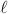=0) carrying optical signals are incident on the DOVG at the angles of its diffraction orders, which are labeled as ±1 and ±2 in Figure 1a. Therefore, along the zeroth order of diffraction, all the incident Gaussian beams are encoded into different OAM channels in the combined coaxial OV beams. Figure 1b1 shows the modeled wavefronts of the OAM with topological charges of=±3 and ±9. Figure 1b2 and 1b3 shows the simulated intensity profiles and wavefronts of the coaxial OV beams. Because of the orthogonal property of OAM, the data carried by the coaxial OV beam can be transmitted in free space without crosstalk among the OAM channels. At the receiver end, all OAM channels are then demultiplexed into Gaussian beams at the corresponding diffraction orders by a second DOVG, which is identical to the grating used for multiplexing. Based on this DOVG-enabled OAM MUX/DEMUX scheme, high-capacity FSO communications can be attained by significantly increasing the number of OAM channels without the need for any additional bulk optics. The uniform power distributions across all the diffraction orders of the DOVGs indicate the high potential scalability of FSO systems with large numbers of high-order OAM channels (Supplementary Information).

We characterize several OAM beams (=±3, ±9, ±15, ±21, ±27) that are generated, multiplexed and demultiplexed by our designed 1×10 DOVGs. Figure 1d1–1d5 shows the intensity profiles of five of the OAM beams (=3, −9, 15, −21, 27) that were captured by an infrared camera at 1550 nm wavelength. These OAM beams have ring-shaped profiles with low intensity at the center and radii proportional to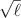, which is consistent with the theoretical results shown in Figure 1e1–1e5. Figure 1d6 shows an image of the combined coaxial OV beam with 10 OAM channels and, for comparison, Figure 1e6 shows the corresponding modeled beam pattern. Figure 1f and 1g shows the measured and modeled intensity profiles of each OAM channel after demultiplexing, respectively. The ring-shaped beams are converted into bright high-intensity spots, which can then be coupled into optical fibers for detection.

Figure 2 shows a schematic diagram of the experimental set-up. The input signals are from a wavelength division multiplexing (WDM) system with 80 wavelengths, spanning from 1529.16 nm to 1560.61 nm with 50 GHz spacing. At each wavelength, the light source is modulated to carry quadrature phase-shift keying (QPSK) signals with 2×50 Gbit s−1 capacities or quadrature amplitude modulation (16-QAM) signals with 2×100 Gbit s−1 capacities, including the multiplexing of the x- and y-polarizations (Supplementary Information). The output from the WDM system is divided equally into 10 branches, which match the diffraction orders of our designed 10-order DOVG. The signals from the 10 branches can be considered to be incoherent and unrelated after propagation in fibers for more than 10 m. These signals are coupled from the fibers to free space with planar phase fronts through fiber collimators. When the beams pass through the DOVG at different angles, the diffraction beams in the zeroth order are loaded by the different OAM channels. We placed a pinhole aperture after the DOVG to filter out the higher orders of the diffracted beams and ensure that only the zeroth order beams can pass through. After propagation in free space over a distance of 1 m, the OV beams with the multiplexed OAM channels are demultiplexed using another grating with exactly the same parameters. When passing through the demultiplexing grating, the coaxial OAM beams are diffracted into 10 orders. The signal beam with a topological charge induced at a certain diffraction angle will be compensated and converted into a Gaussian beam with a bright high-intensity spot at its center. Beams with other topological charges will have ring shapes with low intensity at their centers. We use additional pinhole apertures to filter out these rings. The focused Gaussian beam is then coupled into a fiber by a microscopic objective and is analyzed using a real-time coherent detection system to measure the BER. The optical signal-to-noise ratio (OSNR) is measured using a spectrometer.

## Results and discussion

We achieve a data capacity of 80 Tbit s−1 by multiplexing 1600 channels of 50 Gbit s−1 QPSK signals at 80 wavelengths with 10 OAM states and two polarizations. It should be noted that in this system, all channels can be individually modulated, detected and simultaneously analyzed in real time.

Figure 3a shows the measured optical spectra for OAM states +27 and −9 at the receiver end. The 80 peaks in each spectrum are the 80 wavelengths multiplexed together. From these spectra, we can calibrate the insertion losses (from the transmitter end to the receiver end) for all 10 OAM channels in the range from 33.22 dB to 34.03 dB, including 10 dB loss per DOVG, 0.5 dB loss per lens and 10 dB loss for the processes of passing through the pinholes and coupling into the single mode fiber. The diffraction losses of the DOVG for the 10 orders are in the 9.5–11.3 dB range. Therefore, we can claim that DOVGs enable relatively uniform power distributions for all OAM channels and relax the power constraints used for high-order OAM multiplexing in conventional diffraction gratings. We also measured the BERs of the transmitted signals for all OAM states under different conditions. Figure 3b1 shows the measured BER curves, which depend on the OSNR, at a wavelength of 1544.3 nm without the WDM system. Because the QPSK modulator/coherent detection system is packaged with polarization multiplexing/demultiplexing, each measured BER is the average value of the x- and y-polarizations. The various OSNR values shown in the measurements are attained by controlling the power of the induced white noise. The data points labeled using black squares are the BERs for the back to back measurements, which means that the data transmission occurs directly from the modulator to the detector without the free space propagation. The data points labeled using red circles are the BERs of the signals transmitted from an OAM channel (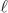=+15) with all other OAM channels switched off. Therefore, in this case, there is no crosstalk between the different OAM channels. The data labeled using blue triangles are the BERs of the signal from the same OAM channel but with all other OAM channels switched on. We see that the data transmissions with all OAM channels available have the highest BER values and gradually approach the values of the case without the OAM crosstalk with increasing OSNR. We attribute this behavior to the superior orthogonal properties of the OAM channels in FSO. With a suitable OSNR, the crosstalk among the different OAM channels can be suppressed. We repeat the BER measurements using an 80-wavelength WDM system as the input light source. Figure 3b2 shows the BER–OSNR curves for=15 at λ=1544.53 nm. We set the enhanced forward error correction (EFEC) threshold as a BER of 2×10−3 and calculated the OSNR penalties for OAM-DM. Without the OAM crosstalk, the penalties for all OAM channels are less than 0.5 dB. Even with the OAM crosstalk, the penalties are still less than 1.5 dB. To further characterize the performance of the WDM/OAM-DM system, we also compare the BERs in the same OAM channel (=−15) at different wavelengths, as shown in Figure 3d. For visualization purposes, we also measured the constellations of the QPSK signals from the demultiplexed OAM beams. Figure 3e1 and 3e2 shows the constellations of the OAM channels=3 and=−21 at a BER of 5×10−4. The similarity of the two figures proves that the performances of the different OAM channels are similar. Figure 3e3 and 3e4 shows the constellations at BERs of 1.2×10−3 and 3.3×10−3 of OAM channel=3. We can see that an overlap appears in the constellation when the BER exceeds the EFEC threshold (2×10−3), which means that the bit error cannot be corrected.

We have demonstrated the OAM-based free-space data link of 1 m length in the proof of concept experiment. To estimate the performance of OAM-based MUX/DEMUX technology for long-distance FSO, we model the OAM beams' propagation in an atmosphere based on the Kolmogorov turbulence theory.29,30,31,32,33 We take the random intensity and phase perturbation of OAM beams into account and calculate the crosstalk among the OAM states. We define Smn as the percentage of beam energy transferred from the OAM state m to n. While m=n, Smn is the power remaining in the original OAM state m; while mn, Smn is the crosstalk between OAM states m and n. We define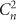as the refractive-index structure parameter with a unit of m−2/3, which is related to the strength of turbulence in the atmosphere.

For=3 as the input OAM state (labeled as Δ=0), we model the Smn parameters of the input OAM state and the nearby states with topological charge differences Δfrom 1 to 6 after 1, 100, 500 and 1000 m propagation in some weak (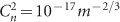) and moderate (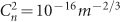) turbulence. Figure 4a shows that there is still 90% energy remaining in the input OAM state after the 1000 m propagation in the atmosphere with the weak turbulence. We also find from the curves that a greater topological charge difference Δgives a smaller energy coupling Smn between the OAM states. For the two adjacent OAM communication channels=3 and=9 in our experiment, labeled as Δ=0 and Δ=6, respectively, the crosstalk is less than 0.2%. According to the modeling results, we claim that the demonstrated OAM based FSO system is robust enough to overcome the crosstalk induced by kilometer-length propagation in the weak turbulence. By contrast, Figure 4b shows that there is only approximately 60% energy remaining in the input OAM state after the 1000 m propagation in the atmosphere with moderate turbulence. In this case, the crosstalk between the input and adjacent OAM channels is above the EFEC threshold and the error bits cannot be corrected. Therefore, under the moderate and strong turbulence conditions, we need to adopt some adaptive optics approaches34 to compensate for the fluctuations of OAM-based long-distance free-space communication.

The DOVG with its pure phase structures is described using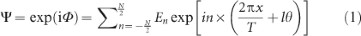where Φ is the phase function, N is the total number of diffraction orders (which is an even positive integer), T is the period of the grating, n is the diffraction order from −N/2 to N/2, θ is the azimuth angle in polar coordinates and l is the interval of the topological charges, which is a non-zero integer. |En|2=1/N is the power of the nth order normalized with respect to the total power. According to the above equation, a beam with a plane wavefront incident on the DOVG is diffracted into N orders, each with equal energy carrying topological charge n×l. As an example, Figure 5a shows the modeling results for OAM beams (=+3, −3, +6 and −6) generated from a Gaussian beam passing through a 1×4 DOVG.

When we implement the DOVG in an inverse manner, multiple OAM beams will be generated and will then be multiplexed into a coaxial OV beam. As shown in Figure 5b, for a beam with a plane wavefront incident from the nth order direction, n=n1 (−N/2≤n1N/2), the diffraction component in the zeroth order diffraction direction will be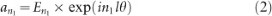With the number of plane waves N incident on the grating from the corresponding diffraction direction of the DOVG, the complex amplitude distribution of the collinear OV beam in the zeroth order direction is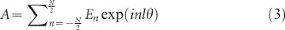Therefore, the multiple incident beams from different angles can synthesize a coaxial beam loaded with different OAM channels using a DOVG and a pinhole filter. OAM multiplexing also introduces an insertion loss of 10×lg(N) dB because the high diffraction orders are all filtered by the pinhole.

We use a DOVG with exactly the same parameters to demultiplex the OAM channels from the coaxial OV beam. When a beam with a specific topological charge (such as n1×l) is incident on the DOVG, the beam can be diffracted into N orders, which can be described as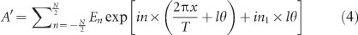The diffraction beam in the −n1th order (i.e., n=−n1) is converted into a plane wave (with topological charge of 0), which can then be focused into a Gaussian-shaped light spot and coupled into an optical fiber for detection while the other diffraction orders are still ring spots, as shown in Figure 5c. We must note that the diffraction energies are equally divided among all designed N orders by the DOVG, which solves the problems of detection of weak signals from the higher orders of a conventional grating. Thus, the dynamic range of the OAM channel detection is improved by the DOVG. Incident coaxial OV beams carrying massive OAM channels can be demultiplexed in parallel by a single DOVG. There is no need for an additional optical splitter or for complex computer-generated holography in the detection system.

In the experiment, we used an optimized binary (0–π) phase vortex Dammann grating for the OAM multiplexing/demultiplexing processes. This binary phase element has the advantages of simple manufacture and low cost. The DOVG not only simplifies the system complexity significantly, but also extends the OAM detection range and increases the system capacity. According to our simulation results, the grating can detect tens or even hundreds of OAM channels simultaneously.

## Conclusions

Since OAM is a new multiplexing technology, it is natural that we question the level to which we can increase the channel numbers while maintaining reasonable performance for practical communication applications. In this article, we report the DOVG-enabled parallel detection of independent QPSK/16-QAM signals from 10 OAM channels as an example to prove our concept. We choose topological charge values of OAM with a relatively large interval of 6 to reduce interference between the collinear OAM channels, where a large OAM dynamic range is demonstrated experimentally. Even with an OAM interval of 1, the measured BERs are still above the EFEC threshold in our communication system. For one-dimensional DOVG, we estimate that several tens of OAM channels can be properly detected with sufficient diffraction angle intervals to arrange the appropriate filters and detectors. If we extend the DOVG to produce a two-dimensional element, the spatial constraints for massive OAM channels detection will be greatly relaxed. For example, we can increase the detection range of the OAM states from ±2 to ±12 with the same diffraction angles by replacing the one-dimensional (1×5) DOVG with a two-dimensional (5×5) element.35 Another advantage of this method is immunity to stringent alignment and phase matching requirements between the light beams and the optical elements.7 This property can be used to simplify the set-up and improve its reliability for FSO communication systems. We therefore believe that the data capacity of FSO communications based on DOVG-enabled OAM-DM can be increased greatly.

## Author contributions

TL, MZ and YL contributed equally to this paper. XY and ZL initiated and directed the project and oversaw the manuscript. TL, MZ, PJ, YL, GNL and XX were responsible for the experimental investigations. CM and JL were responsible for the theoretical studies. CY and HN were responsible for optical and communication systems.

## Conflicts of interest

The authors declare no competing financial interests. Correspondence and requests for materials should be addressed to XY and ZL.